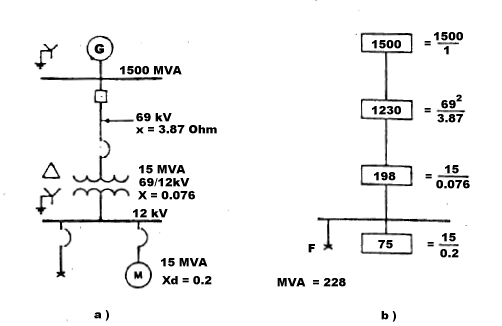## What is the MVA method for short circuit analysis?

#### Back to F.A.Q.

The MVA method is a modification of the Ohmic method where the impedance of a circuit equals the sum of the impedances of components constituting the circuit. Using the admittances, it follows that the reciprocal of the system impedance is the sum of the reciprocals of the admittances of the components. By very definition, the circuit component admittance is the maximum current or KVA at unit voltage which would flow through the circuit or component to a short circuit or fault when supplied from a source of infinite capacity. In practice, the MVA method is used by separating the circuit into components and calculating each component with its own infinite bus as shown in figures a) and b) below:Figure a) is a typical impedance diagram of a one line diagram. Figure b) is an MVA diagram. The conversion from impedance diagram (part a ) to MVA diagram (part b ) is a simple arifmetic. The 1500 MVA power supply is merely given a short circuit MVA rating. Sometimes, if the system MVA is not available, but its voltage and impedance are given, the short circuit MVA can be calculated by MVASC = KV2 * Y formula. The very same formula is used to calculate the short circuit MVA rating of the 69 kV X=3.87 Ohm cable. Next, for the 69/12kV X=0.076 p.u. 15 MVA transformer use MVASC = MVA / Zp.u. formula. The short circuit MVA contribution of the 15 MVA Xd=0.2 motor is equal to its own MVA base divided its own per unit impedance. As a conversion is being made, an MVA diagram is being developed. One line diagram a) is replaced with MVA diagram b). If a short circuit is taken at the 12 kV bus, there will be a series flow of MVA=1500, MVA=1230 and MVA=198, and their combination will be in parallel with the motor SC MVA=75. Combined MVA of components connected in series and parallel are calculated using following equations:

series
MVA1, 2 = MVA1 * MVA2 / (MVA1 + MVA2)
parallel
MVA1, 2 = MVA1 + MVA2
Equations 1

As can be seen from the formulas above, series MVAs are being calculated same as resistances in parallel. Parallel MVA combinations are done same as resistances in series. MVA diagram undergoes same reduction process as impedance diagram by only exeption that MVA values are used instead of per unit impedances or reactances. In our example, MVA1,2 = 1500 * 1230 / (1500 + 1230) = 675. This is new MVA1. MVA1,3 = 675 * 198 / (675 + 198)= 153. MVA1+4 = MVA1 + MVA4 = 153 + 75 = 228. Once the short circuit MVA is found, short circuit current value can be determined using IF, kA = MVAF / (1.73 * KV) formula.

The above approximation is recognized and widely utilised by industry in calculating power system short circuits where the reactance of all circuit components far exceeds resistance producing a consistently high X/R ratio throughout system. The MVA method can be further extended to perform vector addition of SC MVA contributed by system equipments with arbitrary X/R ratios at every point where they intersect. This provides an extremely accurate analysis of the maximum short circuit MVA any node can be subject to. When doing the calculations by hand, use the formulas below to calculate total MVA of components connected in series and parallel with the X/R adjustments:

series
MVA1, 2 = MVA1 x MVA2 x A21 x A22 / [ MVA21 x A21 x A22 + MVA22 x A22 x A21 + 2 x MVA1 x MVA2 x A1 x A2 x (1 + (X/R)1 x (X/R)2) ]1/2
(X/R)1,2 = (MVA1 x A1 x (X/R)2 + MVA2 x A2 x (X/R)1) / (MVA1 x A1 + MVA2 x A2)
where A1 = (1 + (X/R)21)1/2, A2 = (1 + (X/R)22)1/2
parallel
MVA1,2 = 1 / Z1,2
Z1,2 = (R1,2 + X1,2)1/2
R1,2 = ( R1x(R22 + X22) + R2x(R21 + X21) ) / ( (R1 + R2)2 + (X1 + X2)2 )
X1,2 = ( X1x(R22 + X22) + X2x(R21 + X21) ) / ( (R1 + R2)2 + (X1 + X2)2 )
where, R1 = 1 / ( MVA1 x (1 + (X/R)12)1/2), R2 = 1 / ( MVA2 x (1 + (X/R)22)1/2)
X1 = R1 x (X/R)1, X2 = R2 x (X/R)2
Equations 2

Note that the above formulas for series and parallel MVA values simplify to Equations 1 when X/R ratios are equal.

The Short-Circuit-Analytic (SCA) software program is based on the MVA method for short circuit analysis and performs available fault currents calculations in three-phase electric power systems you are working with. The program takes into consideration electrical parameters of the power supply as well as the power distribution system including utility, cables, bus ducts, transformers, generators, motors etc. SCA automatically converts the entire system into a unique impedance unit from which the short circuit current at each and every point is calculated. The process is simple, efficient and will save you both money and time.

Recommended reading: Short Circuit ABC - Learn It in an Hour, Use It Anywhere, Memorize No Formula article by Moon H. Yuen.

4.00

4.00

10/15/2015

09/25/2015

08/18/2015

04/06/2015

11/23/2014

10/16/2014

09/23/2014